1800-1023-196

+91-120-4616500

# Molarity

## Molarity (M)

Molarity of a solution is the number of moles of the solute per litre of solution (or number of millimoles per ml. of solution). Unit of molarity is mol/litre or mol/dm3 For example, a molar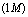solution of sugar means a solution containing 1 mole of sugar (i.e., 342 g or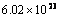molecules of it) per litre of the solution. Solutions in term of molarity generally expressed as,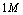= Molar solution,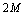= Molarity is two,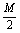or 0.5 M = Semimolar solution,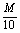or 0.1 M = Decimolar solution,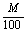or 0.01 M = Centimolar solution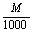or 0.001 M = Millimolar solution

Molarity is most common way of representing the concentration of solution.

· Molarity is depend on temperature as,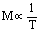· When a solution is diluted (x times), its molarity also decreases (by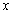times)

Mathematically molarity can be calculated by following formulas,

(i)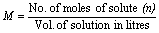,

(ii)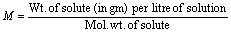(iii)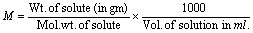(iv)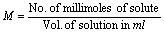(v)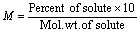(vi)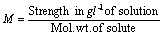(vii)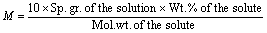(viii) If molarity and volume of solution are changed from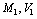to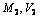. Then,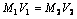(Molarity equation)

(ix) In balanced chemical equation, ifmoles of reactant one react with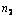moles of reactant two. Then,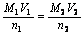(x) If two solutions of the same solute are mixed then molarity (M) of resulting solution.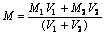(xi) Volume of water added to get a solution of molarity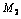from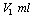of molarity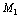is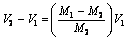## NEET & AIIMS Exam Sample Papers

 AIIMS SAMPLE PAPERS View More NEET SAMPLE PAPERS View More